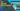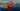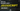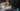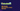# Excel Formulas and Functions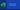Excel formulas and functions are two words that you may have heard a lot during the Excel learning process. Those words are sometimes used interchangeably, this is why they might be confusing at times. Do not worry we are here to make you understand their difference with examples.

## What is a Function?

A function is an expression that is predefined by Microsoft to perform a specific task. The function has a name that ends with Parentheses () in the end.

Between these parentheses, we pass on the parameters into the formula.

### Example

RAND() is a function that is predefined in excel to print a number randomly between 0 to 1.

``````=RAND() // 0.892964198
``````

There is not much, this function can do. It is just designed to print the numbers between 1 and 0. This is why it is called a function.

### Example 2

Randbetween() is a function to print the numbers between two specific numbers. This function takes two numbers as parameters and prints the number between the parameters.

``=RANDBETWEEN(10,20) // 17``

In the function above, the function is taking 10 and 20 as parameters so it will give us the number between 10 and 20 every time the sheet refreshes.

Note – Both Rand() and Randbetween() change their values when the sheet refreshes. We will have to fix their values if we don’t want them to change.

## What is a Formula?

A formula in Excel is an expression that is not pre-defined. A user creates a formula as per his or her own need. A user may or may not use predefined functions in a formula.

## Why Formula?

Microsoft has been working hard to provide us pre-defined functions for all of our needs but they cannot predefine a function for everything because our needs are endless and unique. This is why they have allowed us to form formulas.

It is like they have provided us with all the ingredients for cooking so that we can prepare our cuisine that suites our taste.

Let me show some examples of formulas.

``=2+2 // Output - 4``
``=322 + 233 // Output - 555``
``=A1+B1+C1+D1 // Output - It will add the numerical values in cell A1, B1, C1 and D1``

In the formulas above we have not used the predefined functions. We have written a formula to add values inside the cells. To add the values we have used plus operator (+). The Plus (+) is a mathematical operator. We are allowed to use all the mathematical and logical operators in the formula.

### Formula with Predefined Functions

We are allowed to use functions in the formulas. Let’s see an example of a formula with predefined functions.

``=TODAY()+2``

In the formula above, we are using the Today() function to print today’s date and then add two days to the current date. For Instance, if today is 22 January 2022, this formula will give the result as 24 January 2022. This formula is written to print a day that is two days ahead. Similarly, we can minus days as well.

Let us see some more complicated formulas.

``=IFERROR(VLOOKUP(\$J\$1&" "&\$J\$2&" "&\$J\$3,\$A\$1:\$F\$12,5,0),"Not Available")``

You can see the above formula is also an example of a formula that uses predefined functions. IFERROR and VLOOKUP are two predefined functions, they are used together to form a formula.

The above formula gives us the qualification from the table based on the first name, last name, and department by using the vlookup function. In case the combination does not match, IFERROR prints the Not Available message inside the cell.

In case you don’t know what is Vlookup and how to use it. We have covered this topic in detail in our last article What is Vlookup and How to use it?

## References in Formula

Microsoft Excel sheet is not a notepad. It is a result of evolution. It can recognize the reference and change the formula when we drag a formula.

Suppose we have five rows with numbers and we want to add them in the last cell of the row. We write the formula for the first row and drag it to the last row. We do not write the formula for each and every row separately.

Microsoft recognise that we want to use the same formula for below rows and update the references in the formula automatically.

Let us see this example.

See the screenshots above, we did not write the formula for all 5 rows. We just dragged the formula of the first row and till the fifth row, the formula is added and updated.

## How to Fix a Reference

In case you do not want the reference to change in a formula, you can fix the reference by using the dollar sign (\$). You can see in the vlookup example, we have fixed the references.

To add the dollar sign to fix a reference. Click on the reference and press f4 on the keyboard. It will add the dollar sign in the reference.

I hope the concept of Excel Formulas and Functions is clear for you now. Feel free to give us feedback or article suggestions.

See you in the next article. Have a good one!

Tags

Search by